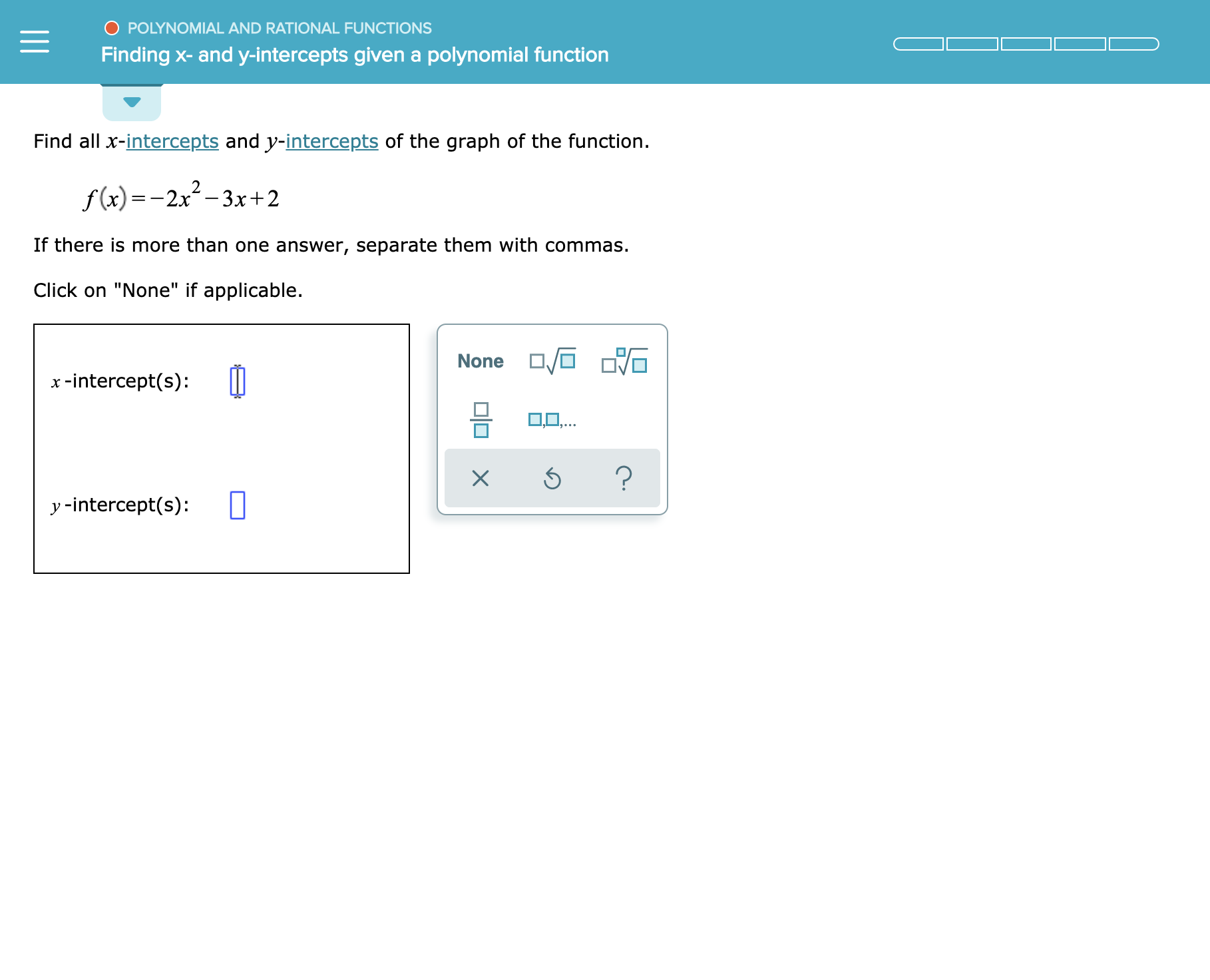O POLYNOMIAL AND RATIONAL FUNCTIONSFinding x- and y-intercepts givena polynomial functionFind all x-intercepts and y-intercepts of the graph of the functionf(x)-2x-3xr+2If there is more than one answer, separate them with commasClick on "None" if applicableNonex-intercept(s)LO..?Xy -intercept(s)

Question

See attachmenthelp_outlineImage TranscriptioncloseO POLYNOMIAL AND RATIONAL FUNCTIONS Finding x- and y-intercepts givena polynomial function Find all x-intercepts and y-intercepts of the graph of the function f(x)-2x-3xr+2 If there is more than one answer, separate them with commas Click on "None" if applicable None x-intercept(s) LO.. ? X y -intercept(s) fullscreen
Step 1

For x intercepts, set f(x) =0 and solve for x

For y intercepts, set x = 0 in f(x) and obtain the value of f(0)

Step 2

x intercept:

y = -2x2- 3x + 2 = 0

Or, -2x2- 4x + x + 2 = 0

Or, -2x(x + 2) + (x + 2) = 0

Or, (1 – 2x)(x + 2) = 0

H...

Want to see the full answer?

See Solution

Want to see this answer and more?

Our solutions are written by experts, many with advanced degrees, and available 24/7

See Solution
Tagged in

Calculus